# Samacheer Kalvi Books: Tamilnadu State Board Text Books Solutions

## Samacheer Kalvi 8th Maths Chapter 4 Life Mathematics Notes PDF Download: Tamil Nadu STD 8th Maths Chapter 4 Life Mathematics NotesSamacheer Kalvi 8th Maths Chapter 4 Life Mathematics Notes PDF Download: Tamil Nadu STD 8th Maths Chapter 4 Life Mathematics Notes

## Samacheer Kalvi 8th Maths Chapter 4 Life Mathematics Notes PDF Download

We bring to you specially curated Samacheer Kalvi 8th Maths Chapter 4 Life Mathematics Notes PDF which have been prepared by our subject experts after carefully following the trend of the exam in the last few years. The notes will not only serve for revision purposes, but also will have several cuts and easy methods to go about a difficult problem.

 Board Tamilnadu Board Study Material Notes Class Samacheer Kalvi 8th Maths Subject 8th Maths Chapter Chapter 4 Life Mathematics Format PDF Provider Samacheer Kalvi Books

## How to Download Samacheer Kalvi 8th Maths Chapter 4 Life Mathematics Notes PDFs?

2. Click on the Samacheer Kalvi 8th Maths Notes PDF.
3. Look for your preferred subject.
4. Now download the Samacheer Kalvi 8th Maths Chapter 4 Life Mathematics notes PDF.

## Tamilnadu Samacheer Kalvi 8th Maths Solutions Chapter 4 Life Mathematics InText Questions

Try These (Text Book Page No. 122)

Find the indicated percentage value of the given numbers.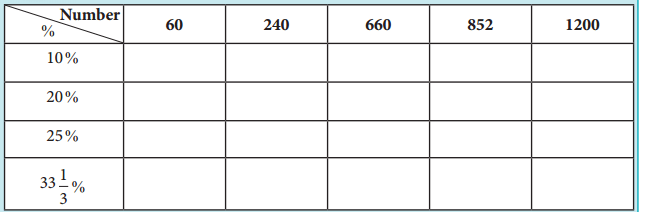Try These (Text Book Page No. 124)

Question 1.
What percentage ola day is 10 hours?
In a day, there are 24 hours .
∴ 10 hrs out of 24 hrs is 1024
As a percentage, we need to multiply by 100
∴ Percentage = 1024 × 100 = 41.67%

Question 2.
Divide ₹ 350 among P, Q and R such that P gets 50% of what Q gets and Q gets 50% of what R gets.
Let R get x, Q gets 50% of what R gets
∴ Q gets = 50100×x=x2
P gets 50% of what Q gets .
∴ P gets = 50100×x2=x4
Since 350 is divided among the three
∴ 350 = x+x2+x4
350 = 4x+2x+x4=7x4 = 350
x = 350×47
Q gets = x2=2002 = 100,
P gets = x4=2004 = 50
∴ p = 50, Q = 100, R = 200

Think (Text Book Page No. 124)

With a lot of pride, the traffic police commissioner of a city reported that the accidents had decreased by 200% in one year. He came up with this number by stating that the increase in accidents from 200 to 600 is clearly a 200% rise and now that it had gone down from 600 last year to 200 this year should be a 200% fall. Is this decrease from 600 to 200, the same 200% as reported by him? Justify.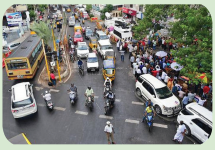Increase from original value 200 to 600Decrease from original value 600 to 200here original value is 600
% decrease = 600−200600 × 100 = 400600 × 100 = 66.67 % decrease
Increase from 200 → 600 and % decrease from 600 → 200 are not the same

Try These (Text Book Page No. 126)

Question 1.
If the selling price of an article is less than the cost price of the article, then there is a ________ .
Loss

Question 2.
An article costing 5000 is sold for ₹ 4850. Is there a profit or loss? What percentage is it?
Loss
Percentage of Loss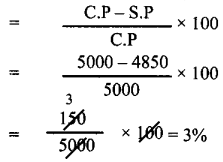Question 3.
If the ratio of cost price and the selling price of an article is 5:7, then the profit / gain is ________ %.
C.P = 5x
S.P = 7x
Profit = 7x – 5x = 2x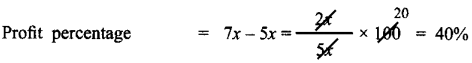Think (Text Book Page No. 129)

A shopkeeper marks the price of a marker board 15% above the cost price and then allows a discount of 15% on the marked price. Does he gain or lose in the transaction?
Let cost price of marker board be 100
CP = 100 Marks it 15% above CP
∴ Marked price MP = 15100 × CP + CP
= 15100 × 100 + 100 = 15 + 100 = 115
Discount % = 15 %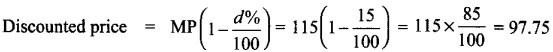∴ He sells it 97.75 which is less than his cost price. Therefore he loses
Loss = 97.75 – 100 = – 2.25

Try These (Text Book Page No. 129)

Question 1.
The formula to find the simple interest for a given principal is ________ .
PNR100

Question 2.
Find the simple interest on ₹ 900 for 73 days at 8% p.a.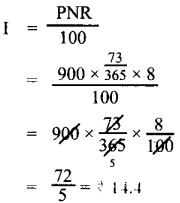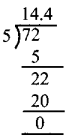Question 3.
In how many years will ₹ 2000 become ₹ 3600 at 10% p.a simple interest?
I = 3600 – 2000 = 1600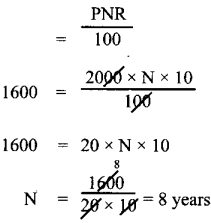Try These (Text Book Page No. 141)

Question 1.
Classify the given examples as direct or inverse proportion:
(i) Weight of pulses to their cost.
As weight increases cost also increases.
∴ Weight and cost are direct proportion.

(ii) Distance travelled by bus to the price of ticket.
As the distance increases price to travel also increases,
∴ Distance and price are direct proportion.

(iii) Speed of the athelete to cover a certain distance.
As the speed increases, the time to cover the distance become less.
So speed and üme are in indirect proportion.

(iv) Number of workers employed to complete a construction in a specified time.
As the number of workers increases, the amount of work become less, so they are in indirect proportion.

(v) Area of a circle to its radius.
If the radius of the circle increases its area also increases.
∴ Area and radius of circles are direct proportion.

Question 2.
A student can type 21 pages in 15 minutes. At the same rate, how long will it take student to type 84 pages?
Direct proportion
No. of minutes = x
k = 2115
2115=84x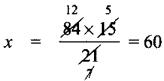Question 3.
If 35 women can do a piece of work in 16 days, In how many days will 28 women do the same work?
Inverse proportion
No. of days = x
k = 35 × 16
∴ 28 × x = 35 × 16Try These (Text Book Page No. 145)

Question 1.
If x and y vary directly, find k when x = y = 5.
I If x andy vary directly then xy = k.
Here x = 5; y = 5
∴ k = 55
k = 1

Question 2.
If x and y vary inversely, find the constant of proportionality when x = 64 and y = 0.75
Gìven x = 64, y = 0.75
and also given x andy vary inversely.
∴ xy = k. the constant of variation.
∴ Constant = 64 × 0.75
Constant of variation = 48

Activity (Text Book Page No. 145)

Draw a circle of a given radius. Then, draw its radii in such a way that the angles between any two consecutive pair of radii are equal. Start drawing 3 radii and end with drawing 12 radii in the circle. List and prepare a table for the number of radii to the angle between a pair of consecutive radii and check whether they are in inverse proportion. What is the proportionality constant?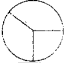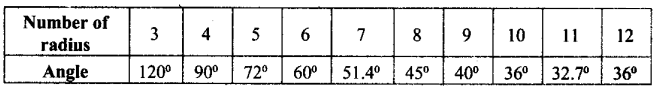As the number of radii increases angle decreases.
Hence they are in inverse proportion
∴ xy = 4 proportional constant
3 × 120° = 360° = k = 360°

Try These (Text Book Page No. 147)

Identify the different variations present in the following questions:

Question 1.
24 men can make 48 articles in 12 days. Then, 6 men can make _____ articles in 6 days.
Let the required no. of articles be x

 Men (P) Days (D) Articles (W) 24 12 48 6 6 x

(i) Mens and days are Indirect variables.
(ii) Men and Articles are direct vanables
(iii) Days and articles are also direct variables using formula.
Let
P1 = 24
P2 = 6

D1 = 12
D2 = 6

W1 = 48
W1 = x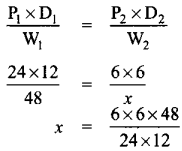x = 6 Articles

Question 2.
15 workers can lay a road of length 4 km in 4 hours. Then, _____ workers can lay a road of length 8 km in 8 hours.
Let the required no. of workers be x

 Length (work) Hours Workers 4 km 4 hrs 15 8 km 8 hrs x

(i) Length and workers are direct variable as more length need more workers.
The proportion is 4 : 8 : : 15 : x ——– (1)
(ü) Hours and workers are indirect variables as more working hours need less men.
∴ The proportion is : 4 : : 15 : x ——– (2)
Combining (1) and (2)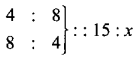Product of the extremes = Product of the mean
4 × 8 × x = 8 × 4 × 15
x = 8×4×154×8
x = 15 workers

Question 3.
25 women working 12 hours a day can complete a work in 36 days. Then, 20 women must work hours a day to complete the same work in 30 days.
Let the required hours be x.

 Women Days Hours 25 36 12 20 30 x

As women increases hours to work decreases
∴ It is an inverse proportion.
∴ Multiplying factor is 2520
As days increases hours needed become less
∴ It is also an indirect variation.
∴ Multiplying factor is 3630
∴ x = 12×2520×3630
x = 18 hours

Question 4.
In a camp there are 420 kg of rice sufficient for 98 persons for 45 days. The number of days that 60 kg of rice will last for 42 persons is .
Let the required number of days be x.

 Rice (kg) Men Days 420 98 45 60 42 x

If amount of rice is more it will last for more days;
∴ It is Direct Proportion
∴ Multiplying factor is 60420
If men increases number of days the rice lasts decreases
∴ It is an inverse proportion.
∴ Multiplying factor is 9842
x = 45×60420×9842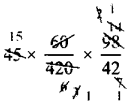x = 15 days

Try These (Text Book Page No. 150)

Question 1.
Vikram can do one-third of work in p days. He can do th of work In ________ days.
13 of the work will be done mp days.
∴ Full work will be completed in 3p days
34 th of the work will be done in = 3p x 34
= 94p = 214 p days.

Question 2.
If m persons can complete a work in n days, then 4m persons can complete the same
work in ______ days and m4 persons can complete the same work in ______ days.
Givenm persons complete a work in n days
(i) Then work measured in terms of Man days = mn
4 m men do the work it will be completed in mn4m days = m4 days.## How to Prepare using Samacheer Kalvi 8th Maths Chapter 4 Life Mathematics Notes PDF?

Students must prepare for the upcoming exams from Samacheer Kalvi 8th Maths Chapter 4 Life Mathematics Notes PDF by following certain essential steps which are provided below.

• Use Samacheer Kalvi 8th Maths Chapter 4 Life Mathematics notes by paying attention to facts and ideas.
• Pay attention to the important topics
• Refer TN Board books as well as the books recommended.
• Correctly follow the notes to reduce the number of questions being answered in the exam incorrectly
• Highlight and explain the concepts in details.

## Frequently Asked Questions on Samacheer Kalvi 8th Maths Chapter 4 Life Mathematics Notes

#### How to use Samacheer Kalvi 8th Maths Chapter 4 Life Mathematics Notes for preparation??

Read TN Board thoroughly, make separate notes for points you forget, formulae, reactions, diagrams. Highlight important points in the book itself and make use of the space provided in the margin to jot down other important points on the same topic from different sources.

#### How to make notes for Samacheer Kalvi 8th Maths Chapter 4 Life Mathematics exam?

Read from hand-made notes prepared after understanding concepts, refrain from replicating from the textbook. Use highlighters for important points. Revise from these notes regularly and formulate your own tricks, shortcuts and mnemonics, mappings etc.
Share: